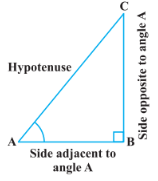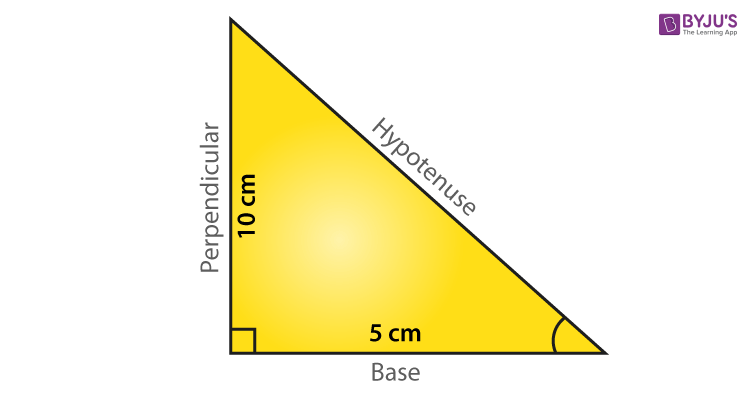# Trigonometry Basics

Introduction to Trigonometry basics is given first in Class 9 to all the students, in their academics. Then, as they move to higher level classes, the concepts of trigonometry are explained more widely. At first, students are taught with basic trigonometry formulas and also to solve problems based on them.

Here in this article, we are going to learn to find trigonometry angles based on formulas and functions. Also, know about different trigonometry ratios such as sine, cosine and tangent of an angle, for a given triangle. There are multiples uses of trigonometry both in academics and in real life. Therefore, students should concentrate while learning about these concepts.

We will study the basics of trigonometry with the help of definitions and examples. Keep reading the article below.

## Trigonometry Definition

Trigonometry is all about triangles. The word ‘Tri’ Greek word which defines as ‘Three’, ‘gon’ means ‘length’ and ‘metry’ is ‘measurement’. So basically in trigonometry basics, we will learn about the measurement of lengths and angles of triangles. Trigonometry is basically used for the right-angled triangle, where one of the angles is 900.

There are basically two branches of trigonometry, one is plane trigonometry and other is Spherical trigonometry.

Plane Trigonometry: To find the relationship between angles and sides of the triangle. Here the sum of interior angles of the triangle is 180°.

Spherical trigonometry: Here, we draw curved triangles on the surface of the sphere, which means the sum of the interior angles is more than 180°. This kind of trigonometry are usually used in navigation.

## Trigonometry Formula

Let us learn, trigonometry basics formula here, taking an example of the right-angled triangle.Consider ABC is a right angled triangle, right angled at B and have one hypotenuse AC and one adjacent AB adjacent to angle A and one perpendicular BC opposite to Angle A.

Trigonometry formulas are based on three major trigonometric ratios, Sine, Cosine and Tangent.

• Sine or sin∠A= $\frac{Perpendicular}{Hypotenuse}$=$\frac{BC}{AC}$
• Cosines or cos∠A= $\frac{Adjacent}{Hypotenuse}$=$\frac{AB}{AC}$]
• Tangent or tan∠A= $\frac{Perpendicular}{Base}$=$\frac{BC}{AB}$

Similarly, we can write the formula for Reciprocal properties, Sec, Cosec and Cot ratios.

• Sec∠A =1/Cos∠A = $\frac{Hypotenuse}{Adjacent}$=$\frac{AC}{AB}$
• Cosec∠A =1/Sin∠A = $\frac{Hypotenuse}{Perpendicular}$=$\frac{AC}{BC}$
• Cot∠A = 1/tan∠A = $\frac{Base}{Perpendicular}$=$\frac{AB}{BC}$

Also,

• Sec∠A .Cos∠A=1
• Cosec∠A .Sin∠A=1
• Cot∠A .Tan∠A=1

Quotient Properties in Trigonometry:

• Tan∠A=Sin∠A/Cos∠A
• Cot∠A =Cos∠A/Sin∠A
• Sin∠A=Tan∠A/Cos∠A
• Cos∠A=Sin∠A/Tan∠A
• Sec∠A=Tan∠A/Sin∠A
• Cosec∠A=Cos∠A/Tan∠A

Some more basic trigonometry formulas:

• Sin(90-∠A)=Cos∠A
• Cos(90-∠A)=Sin∠A
• Tan(90-∠A)=Cot∠A
• Cot(90-∠A)=Tan∠A
• Sec(90-∠A)=Cosec∠A
• Cosec(90-∠A)=Sec∠A

Pythagorean formulas for trigonometry:

• sin2∠A + cos2∠A = 1
• tan2∠A + 1 = sec2∠A
• cot2∠A + 1 = cosec2∠A
• sin2∠A = 2 sin∠A.cos∠A
• cos2∠A = cos2∠A – sin2∠A
• tan2∠A = 2tan∠A/(1 – tan2∠A)
• cot2∠A=(cot2∠A – 1)/ 2cot∠A

## Trigonometry Table

 Angle 0° 30° 45° 60° 90° Sin$\angle$A 0 1/2 $1/\sqrt{2}$ $\sqrt{3}/2$ 1 Cos$\angle$A 1 $\sqrt{3}/2$ $1/\sqrt{2}$ 1/2 0 Tan$\angle$A 0 1/$\sqrt{3}$ 1 $\sqrt{3}$ Undefined Cot$\angle$A Undefined $\sqrt{3}$ 1 $\sqrt{3}$/2 0 Sec$\angle$A 1 2/$\sqrt{3}$/2 $\sqrt{2}$ 2 Undefined Cosec$\angle$A Undefined 2 $\sqrt{2}$ 2/$\sqrt{3}$/2 1

### Trigonometry Problem

With the above-given formulas and identities, we can solve trigonometry basics problems very easily.

Example: If a triangle ABC has sides, right angled at B, has perpendicular as 10cm and base as 5cm. Find the tangent and cotangent of the angle formed at point A.Solution: we know,

Tan∠A = Perpendicular/Base

Tan∠A = 10/5

= 2 unit

And,

cot∠A = 1/Tan∠A

=1/2

This way you can solve many trigonometric problems, based upon the trigonometric functions, identities and formulas. Also, learn trigonometry basics class 10th problems by clicking on the links given at the end of this article.

Keep learning with us and download BYJU’S- The learning App, for interactive videos.

 MATHS Related Links Trigonometry Angles Trigonometry Trigonometric Identities Trigonometry Formulas Trigonometry Values Trigonometry Graphs